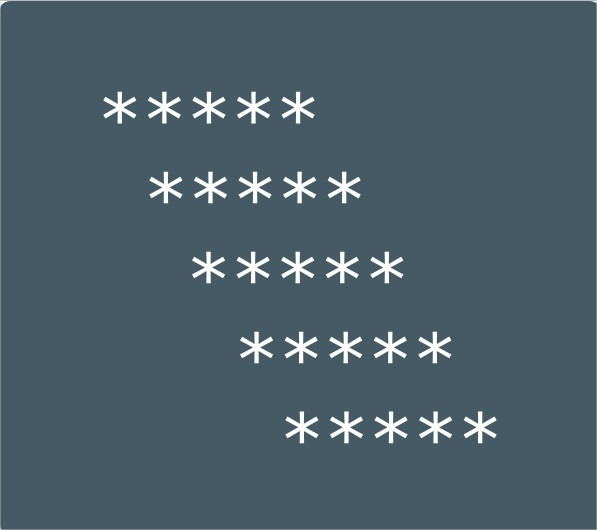# Mirrored Rhombus Star Pattern Program In c | Patterns

C Program to print a Mirrored Rhombus Star Patter – In this particular article, we will brief in on the various methods used to print a Mirrored Rhombus Star Pattern in C Programming.

The ways in which the Mirrored Rhombus Star Pattern is printed in this article are as follows:

• Using For Loop
• Using While Loop
• Using Do-While Loop

As we all know, a Rhombus is a quadrilateral which is bound in nature and has sides of equal length. The opposite sides of a rhombus are parallel to each other.

The opposite angles of a rhombus are also equal in nature. Due to all these characteristics, the diagonals of a rhombus intersect each other at right angles.

In this piece, students have to print a star pattern. Star patterns are basically the introductory level of teaching logic and how to use it in the art of programming.

The Mirrored Rhombus Star Pattern looks like this:As you can see, this is how a Mirrored Rhombus Star Pattern should look like. To print this in C Programming, you will have to check the whole article as you have no other choice right now after clicking this.

Also, check out the sample outputs and sample programs. At the end of the post, we do list out the more C programs and also online execution tool for you. Do check it out.

## Using For Loop

1) Read the no.of rows, store the value into the variable n, read the entered symbol and store it in the variable ch.

2) The outer for loop iterates through rows from i=1 to n with the structure for(i=1;i<=n;i++).

3) The 1st inner for loop iterates through columns from j=i to 1 with the structure for(j=i;j>0;j–).

a) Prints space.

4) For i=1 or n(1st row or nth row)

a) The 2nd inner for loop iterates through columns with the structure for(j=1;j<=n;j++) and prints symbol.

5) For i!=1 or i!=n(other than 1st row,nth row)

The 3rd inner for loop iterates through columns with the structure for(j=1;j<=n;j++)

a) prints symbol if j=1 or j=n

b) Otherwise it prints space.

6) The cursor comes to next line for each iteration.

Output:

### Using While Loop

1) The outer while loop iterates through rows until the condition i<=n becomes false.

2) The 1st inner while loop iterates through columns and prints space until the condition –j>0 becomes false.

3) For i=1 or n(1st row,nth row)

The 3rd inner while loop iterates through columns until the condition j<=n

a) Prints symbol.

4) for i!=1 or i!=n(for other than 1st row,nth row)

The 4th inner while loop iterates through columns until the condition j<=n becomes false.

a) Prints symbol if j=1 or j=n

b) Otherwise it print space.

5) For each iteration of the outer while-loop cursor comes to next line.

Output:

#### Using Do-While Loop

1) For i=1 the outer do-while loop iterates the loop one time through rows.

2) a) For j=i the 1st inner do-while loop iterates through columns and print one space.

b) j value decreased by 1.

c) Checks the condition –j>0. If condition true loop iterates again.Repeats until the condition becomes false.

3) a) For j=1 the 2nd inner do-while loop iterates through columns and prints one character.

b) j value increased by 1.

c) Checks the condition ++j<=n.If the condition is true loop iterates again. Repeat until the condition becomes false.

4) Cursor comes to next line.

5) a) i value increased by 1.

b) Checks the condition i<=n.If the condition is true then outer do-while loop iterate again.Repeats until the condition becomes false.

Output:

x

## C Program To Print Number Of Days In A Month | Java Tutoring

C program to input the month number and print the number of days in that ...